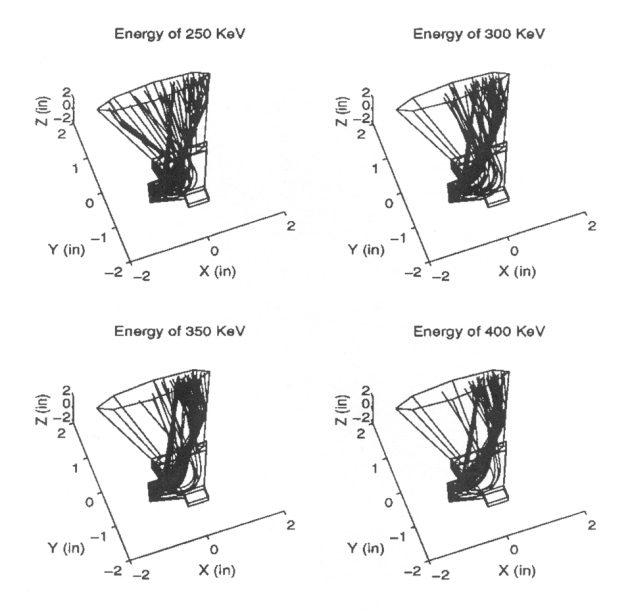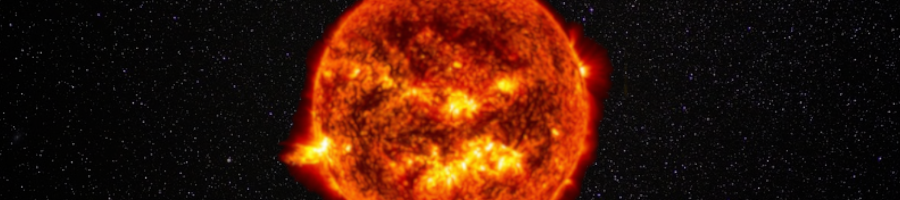ULYSSES

Ulysses HISCALE Data Analysis Handbook

## Appendix 10. Effect of Backscattered Electrons on the Geometric Factors of the LEMS30 Telescope (Hong MS Thesis)

### A10.10 Appendix - Trajectories of the Escaped Electrons (continued)

Figure A10-19a View angle of q = 0º and f = 90º. Neubert's backscattering coefficients are used.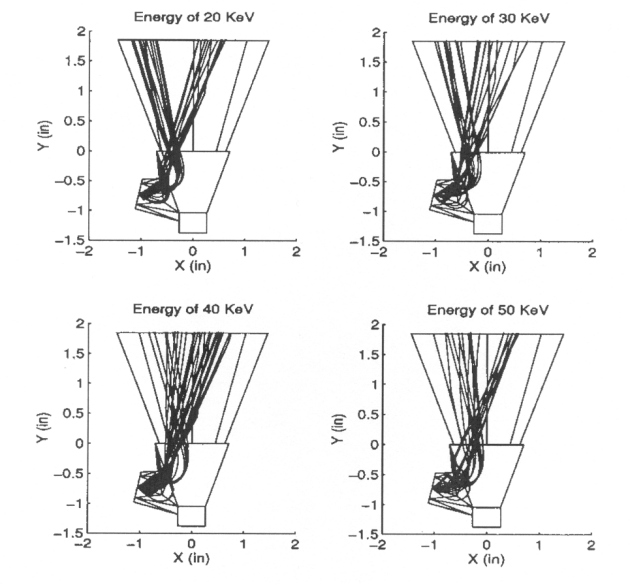Figure A10-19b View angle of q = 0º and f = 90º. Neubert's backscattering coefficients are used.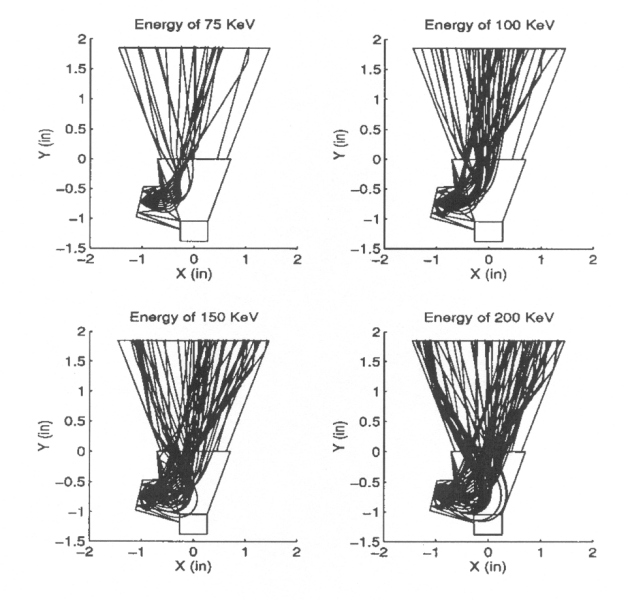Figure A10-19c View angle of q = 0º and f = 90º. Neubert's backscattering coefficients are used.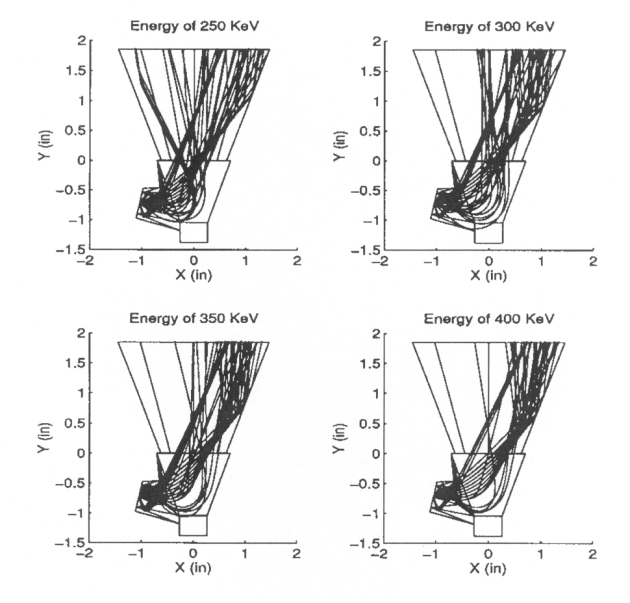Figure A10-20a View angle of q = -20º and f = 80º. Neubert's backscattering coefficients are used.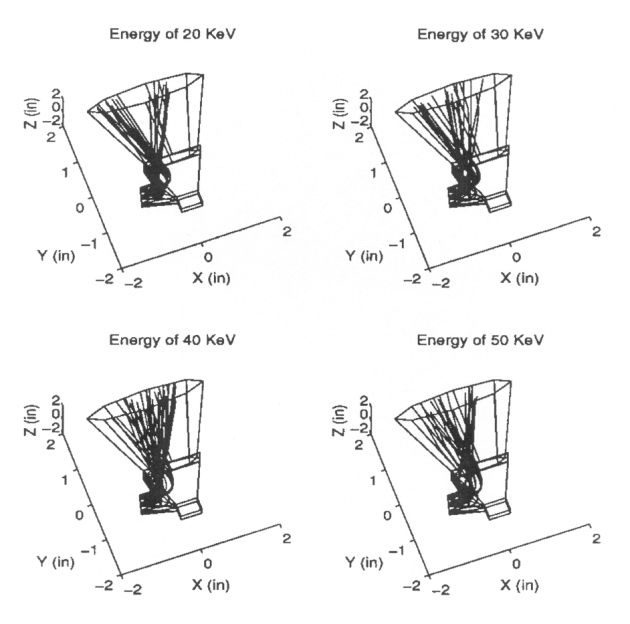Figure A10-20b View angle of q = -20º and f = 80º. Neubert's backscattering coefficients are used.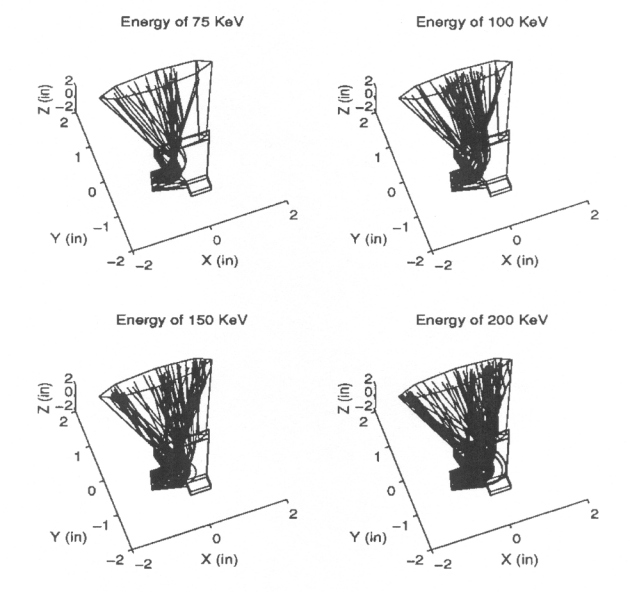Figure A10-20c View angle of q = -20º and f = 80º. Neubert's backscattering coefficients are used.